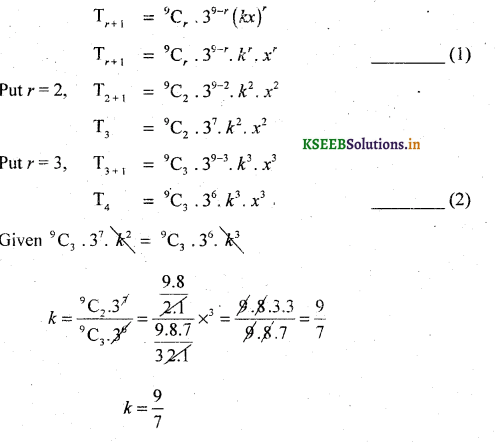# 2nd PUC Basic Maths Question Bank Chapter 4 Binomial Theorem

Students can Download Basic Maths Question Bank Chapter 4 Binomial Theorem Questions and Answers, Notes Pdf, 2nd PUC Basic Maths Question Bank with Answers helps you to revise the complete Karnataka State Board Syllabus and to clear all their doubts, score well in final exams.

## Karnataka 2nd PUC Basic Maths Question Bank Chapter 4 Binomial Theorem

### 2nd PUC Basic Maths Binomial Theorem Two Marks Questions and Answers

Question 1.
Find the 5th term in $$\left(\frac{4 x}{5}-\frac{5}{2 x}\right)^{8}$$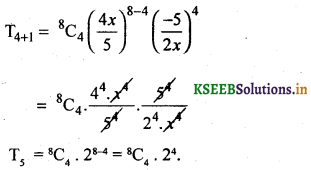Question 2.
Find the 6th term in $$(\sqrt{x}-\sqrt{y})^{17}$$Question 3.
Find the 8th term in $$\left(\frac{a}{2}-\frac{3}{b}\right)^{10}$$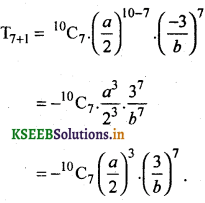Question 4.
Find the 7th term $$\left(3 x^{2}-\frac{y}{3}\right)^{9}$$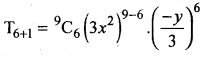Question 5.
Find the 10th term in the Expansion of $$\left(\frac{a}{b}-\frac{2 b}{a^{2}}\right)^{12}$$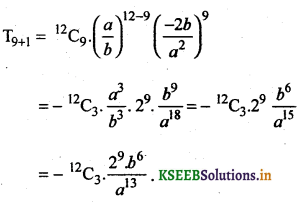### 2nd PUC Basic Maths Binomial Theorem Three or Four Marks Questions and Answers

A Binomial Expansion Calculator that doesn’t require any scripting in your browser. Just enter your values and compute.

Question 1.
Expand (1 – 2x)s using binomial theorem. x = 1, a = – 2x, n = 5.
(1 + (-2x))5 = 15 + 5C1 . I4 (-2x) + 5C2. I3 (-2x)2 + 5C3. I2(-2x)3 + 5C4 . 1(-2x)4 + 5C5(-2x)5
= 1+5 (-2x) + 10(-2x)2 + 10 (-2x)3+ 5 (-2x)4 + (-2x)5
= 1 – 10x + 10 C4x2 + 10(-8x3) + 5 (16x4) + (-32x5)
= 1 – 10x + 40x2– 80x3 + 80x4– 32x5Question 2.
Expand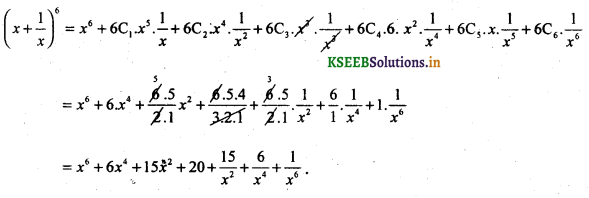Question 3.
Simplify : $$(2+\sqrt{5})^{4}+(2-\sqrt{5})^{4}$$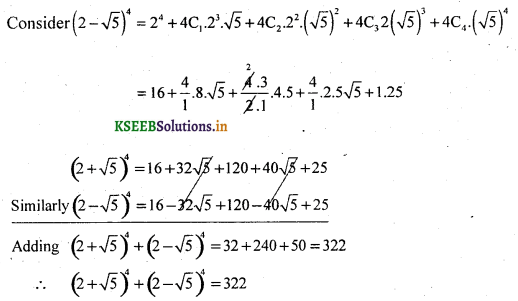Question 4.
Simplify : $$(3+\sqrt{2})^{4}-(3-\sqrt{2})^{4}$$ using Binomial theorem.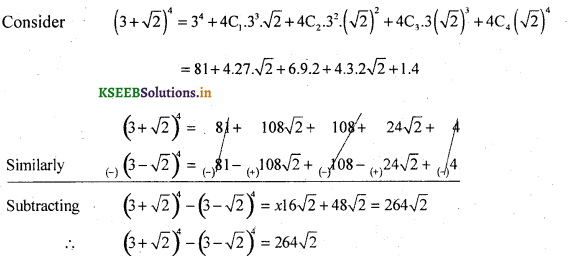Question 5.
Simplify  $$(\sqrt{2}+1)^{6}-(\sqrt{2}-1)^{6}$$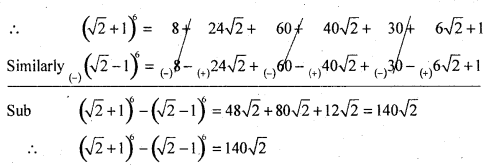Question 6.
Expand (0.99)5 by Binomial theorem correctly decimal places.
(0.99)5 = (1- 0.01)5=15+5C1.14.(- 0.01) + 5C2.13(-0.01)2+5C3.12(-0.01)3+5C4.11(-0.01)4+  5C5 ( – 0:01 )5Question 7.
Expand (0.98)s by Binomial theorem.
(0.98)5 = (1 – 0.02)5
= 15 + 5Cr 14 (- 0.02) + 5Cr 13( – 0.02)2 + 5Cr 12( – 0.02)3 + 5C4. 1(- 0.02)4 + 5C5(- 0.02)5 = 1 – 5(0.02) + 10(0.0004) – 10 (0.000008) + 5(0.00000016) – 0.0000000032
= 1-0.1 + 0.004 – 0.00008 + 0.00000080 – 0.0000000032
= 1.0040008 – 0.1000800032 = 0.9039.

Question 8.
Find the value of (1.02)4 using the binomial theorem.
(1.02)4 =(1 +0.02)4
= 14 + 4C1.13(0.02) + 4C2.12(0.02)2 + 4Cr 1(0.02)3 + 4C4(0.02)4
= 1 +4 (0.02) + 6 (0.0004) + 4 (0.000008) + 1 .(0.00000016)
= 1 + 0.08 + 0.0024 + 0.000032 + 0.00000016
= 1.08243216.Question 9.
Write down the middle term in the expansion of $$\left(2 x^{2}-\frac{1}{x}\right)^{12}$$
Here n = 12,
∴ There are 13 terms. The middle terms is $$\frac{12}{2}+1$$ = 7th terms
To find 7th term put r = 6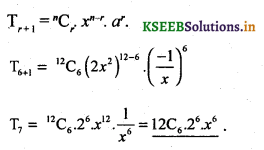Question 10.
Find the middle term of the Expansion of $$\left(\frac{1}{x^{2}}+\frac{x}{2}\right)^{10}$$
Here n = 10, There are 11 terms.
∴  Middle term = $$\frac{10}{2}$$+1 = 6 terms
To find 6th term put r = 5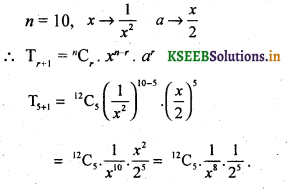Expanding binomials calculator using the binomial expansion method step-by-step.

Question 11.
Find the 5th term in the expansion of $$\left(2-\frac{1}{x}\right)^{3}$$Question 12.
Find the 6th term in the expansion of $$\left(3+\frac{2}{x}\right)^{10}$$Question 13.
Find the value of (10.1)5 up to 4 decimal places, using binomial theorem
(10 + 0.1)5= 105 + 5Cr104.(0.1) + 5C2.103. (0.1)2 + 5Cr102.(0.1)3 + 5C4.10.(0.1)4+5C5(0.1)5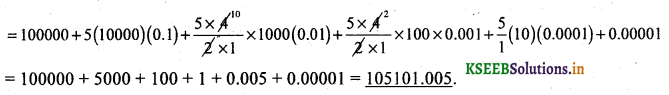Question 14.
S.T there is no term containing x3 in the expression $$\left(3 x-\frac{1}{2 x}\right)^{8}$$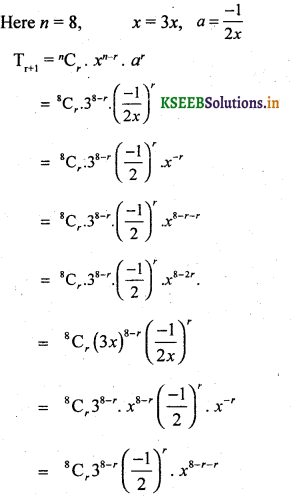To find the term containg x3 equate the power of x to 3
∴ 8 – 2r = 3 ⇒ 5 = 2r = s.r = r = 5 /2
Since r is a fraction there is no term containing x3.

Question 15.
S.T. There is no term independent of x in the expansion of $$\left(2 x-\frac{1}{x^{2}}\right)^{10}$$To find the terms independent of x equate the power of x to O, we get 10 – 3r = 0
⇒ 10 = 3r ⇒ r = $$\frac{10}{3}$$ is a fraction. Hence there is no terms independent of x.

Question 16.
Find the co-efficient of x1 in the expression of $$\left(x^{2}+\frac{2}{x}\right)^{11}$$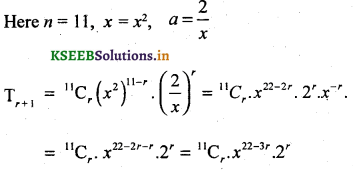To find the coeffecint of x1 equate the power of x to 7
∴ 22 – 3r = 7 ⇒ 22 -1 = 3r ⇒ 15 – 3r  ⇒ r = 5.
∴ Co-efficient of x7 is 11C5.25.

Question 17.
$$\left(\frac{a}{x}+b x\right)^{12}$$
∵  n = 12, we have 13 terms,
∴ 7th term is the middle tem
To find 7th term put r = 6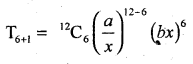Question 18.
$$\left(\frac{a}{3}+\frac{b}{2}\right)^{8}$$
Here n = 8,
∵  we have only one middle term, i.e. 5th terms.
To find 5th term put r = 4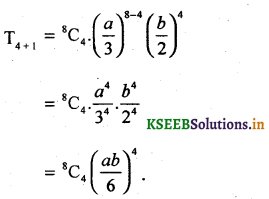Question 19.
$$(3+\sqrt{2})^{6}-(3-\sqrt{2})^{6}$$Question 20.
Find x if the 17th and 18th terms of the expansion (2 + x)50 are equal.
17th term = T16 + 1 = 50C17 . 250 – 16. x16 = 50C16 . 234. x16
18th term = T17 + 1 = 50C17 . 550 – 17. x17 = 50C17 . 233 . x17Question 21.
If 2nd 3rd and 4th terms of (x + y)n are 108,54,12 respectively. Find the value of x,y and n.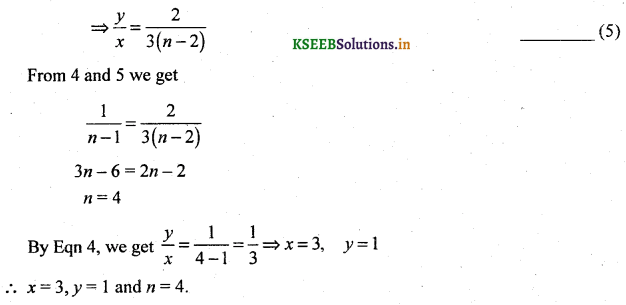Question 22.
If the coefficient of (2r + 4)th and (r – 2)th terms in the expansion of (1 + x)18 are equal then find r.
Tr+1 = 18Cr . (1)18 – r . xr
= 18Cr. xr
Here The coefficient of (r + 1 )th term is 18Cr
Therefore the coefficient of (2r + 4))th term is 18C2r+J
Therefore the coefficient of (r – 2))th term is 18Cr – 3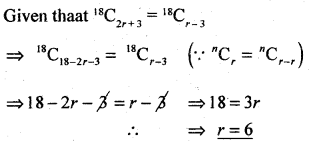Question 23.
Find the middle terms in the expansion of
(i) $$\left(3 x-\frac{2}{x^{2}}\right)^{15}$$
∵ n = 15, we have 2 middle terms i.e., 8th and 9th terms,
(a) To find 8th term put r = 7Question 24.
$$\left(\frac{x}{2}+\frac{3}{x^{2}}\right)^{19}$$
n = 19, (odd no.)
We have two middle terms i.e., 10th and 11th terms,Question 25.
$$\left(2 x^{2}+\frac{1}{\sqrt{x}}\right)^{11}$$
n = 19, (odd no.). We have two middle terms i.e., 10th and 11th terms,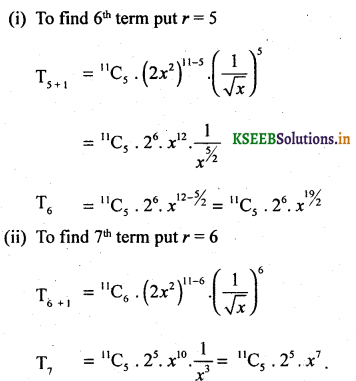Question 26.
Find the coefficients of
(a) $$x^{11} \text { in }\left(x+\frac{2}{x^{2}}\right)^{17}$$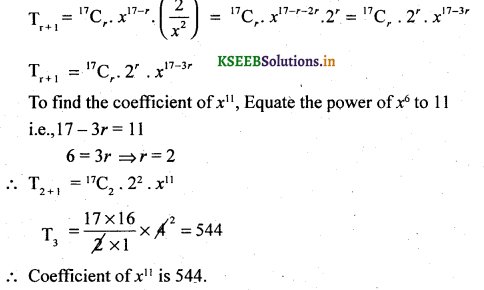Question 27.
Find the coefficient of y2 in $$\left(7 y^{2}-\frac{2}{y}\right)^{12}$$Question 28.
Find the coefficient of y11 in $$\left(\sqrt{x}-\frac{2}{x}\right)^{17}$$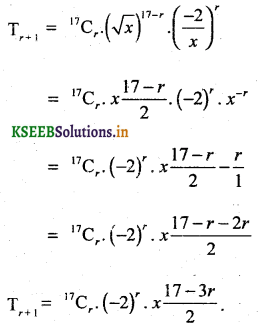Question 29.
To find coefficient of x-11, equate the power of x, to -11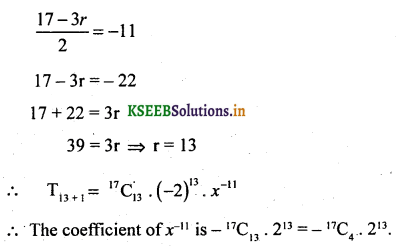Question 30.
Find the term independent of x in.
(i) $$\left(\frac{4 x^{2}}{3}+\frac{3}{2 x}\right)^{9}$$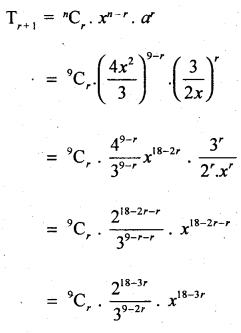To find the term independent of x, equal the power of x to 0
18 – 3r = 0 ⇒ r = 6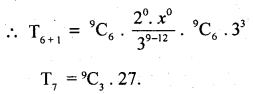Question 31.
$$\left(x^{3}-\frac{3}{x^{2}}\right)^{15}$$To find the term independent of x, equale the power of x to 0
45 – 5r = 0 ⇒ r = 9Question 32.
$$\left(\sqrt{x}+\frac{1}{3 x^{2}}\right)^{10}$$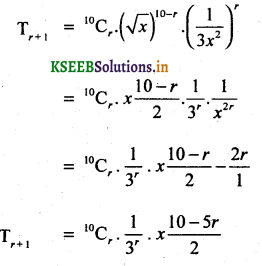To find the terms independent of x , Equale the power of x to 0Question 33.
The first 3 terms in (1 + ax)n where n is a positive integer are 1,6x, 6×2. Find the values of a and n.